## Example Questions

### Example Question #2 : Percent Of Change

The price of a particular metal raises from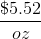to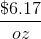. What percent increase did the price see?

Possible Answers: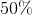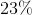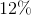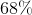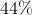Correct answer:Explanation:

To find percent change, subtract the "old" amount from the "new" amount, then divide the result by the "old" amount. The result is a percent increase if positive and a percent decrease if negative.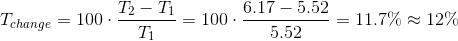### Example Question #51 : Percentage

A particular stock gainson its price on Tuesday. On Wednesday, it losesof its new price. On Thursday, it gains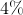on Wednesday's price. What is the percent increase at the close of business Thursday, compared to the opening price on Tuesday? Do not round until the final answer.

Possible Answers: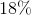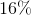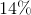Correct answer:Explanation:

To find percent change, subtract the "old" amount from the "new" amount, then divide the result by the "old" amount. The result is a percent increase if positive and a percent decrease if negative. In this case, to keep our amount constantly compared to Tuesday, set Tuesday's price as a variable.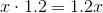On Tuesday, gain 20 percent.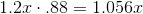On Wednesday, lose 12 percent (thus, multiply by 88 percent).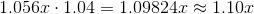On Thursday, gain 4 percent.

Thus, our final gain relative to Tuesday is.

### Example Question #51 : Percentage

What is the percent increase from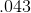to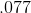?

Possible Answers: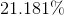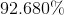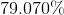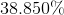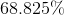Correct answer:Explanation:

To find percent change, subtract the "old" amount from the "new" amount, then divide the result by the "old" amount. The result is a percent increase if positive and a percent decrease if negative.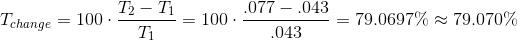### Example Question #11 : Percent Of Change

A fundraiser for a local charity raised $28,000 in donations last year. The fundraiser’s goal for this year is to exceed last year’s donations by 15%. So far, they have raised$31,000. How much more money must the fundraiser raise to reach their goal?

Possible Answers:

$3200$4200

$5500$1200

$1500 Correct answer:$1200

Explanation:

First, let's calculate the value of this year's expected goal. Start by calculating 15 percent of last year's goal. Do this by converting 15 percent to a decimal by moving the decimal point two spaces to the left, and then multiplying this number by last year's goal.Now, we can add this value to last year's goal to obtain the expected goal for this year's fundraiser.Since $31,000 has been raised so far, the amount remaining is as follows:### Example Question #1 : How To Find The Percent Of Decrease What is the percentage decrease from 2.4 gal. to 3.5 qts.? Possible Answers: 63.54 112.53 64.53 33.45 Correct answer: 63.54 Explanation: Find a common unit – in this case it is ounces. The decrease is from 307.2 oz. to 195.2 oz. (128 oz x 2.4 gal. – 32 oz. x 3.5 qts.) 307.2 oz. – 112 oz. = 195.2 oz. Then, 195.2/307.2 = 63.54 % ### Example Question #1 : How To Find The Percent Of Decrease The regular price for a sweater in October is$80. In November, the store holds a Thanksgiving sale, and the price of the sweater is reduced by 7%. In December, the store holds a Christmas sale, and the November price of the sweater is reduced by an additional 6%. At the end of December, Jim purchases the sweater. By what percent did the price of the sweater decrease from October to December?

Possible Answers:

7.2%

11%

12.6%

21%

8.4%

Correct answer:

12.6%

Explanation:

In October, the sweater cost $80. In November, it was reduced by 7%. 7% of$80 is $5.60 (0.07 x 80). Thus, the new price of the sweater is$80-$5.60, which is$74.40. In December, the sweater's price is reduced by an additional 6%. 6% of $74.40 is$4.46 (0.06 x 74.40). Thus, the December price for the sweater is $74.40-$4.46, which equals $69.94. To find the percent change, we take the change ($80-$69.94=$10.06) and divide by the initial price ($80). We then multiply that quotient by 100 to get 12.6%. ### Example Question #51 : Percentage A local appliance store puts a washing machine that usually sells for$200 on sale for \$150.  What is the percent discount?

Possible Answers:

50%

35%

None of the answers are correct

25%

10%

Correct answer:

25%

Explanation:

% Discount = Amount of Discount ÷ Original Amount

% Discount = (Old Price – New Price) ÷ Original Amount

For this problem we have (200 – 150) ÷ 200 = 0.25 or 25% discount

### Example Question #141 : Arithmetic

A company’s annual sales are as follows: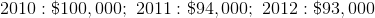What is the percentage decrease in annual income from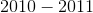and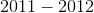?

Possible Answers: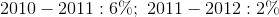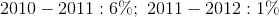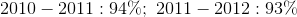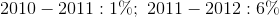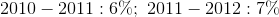Correct answer:Explanation:

2010–2011:

% Decrease = (2010 Income – 2011 Income) / 2010 Income

= (100000 – 94000) / 100000 = 6000 / 100000 = 0.06 = 6%

2011–2012:

% Decrease = (2011 Income – 2012 Income) / 2011 Income

= (94000–93000) / 94000 = 1000 / 94000 = .01 = 1%

### Example Question #1 : How To Find The Percent Of Decrease

In 2014, a basketball team wongames. In 2013, the team wonfewer games than it did in 2014. How many games did the team win in 2013?

Possible Answers:Correct answer:Explanation:

If the team wonfewer games, subtractoffrom:

Step 1: Find% of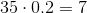Step 2: Subtract from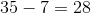### Example Question #11 : Percent Of Change

If a new television cost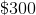for a store to purchase and was originally on sale for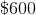, by what percentage must it be marked down in order for the store to make aprofit?

Possible Answers: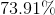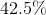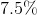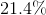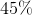Correct answer:Explanation:

First, calculate the amount of profit that will need to be made to earn aprofit. This is done by convertingto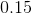and multiplying it byto get. This means that the store can mark down the television to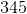dollars. Thus, we are asking for the percent change from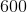to.  This is calculated: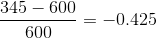This means that it is amarkdown.

### All ACT Math Resources